# Qubit, An Intuition #1 — First Baby Steps in Exploring the Quantum World

The beauty of Quantum Mechanics for Quantum Computation, featuring IBM Quantum

`TL;DR; - The intuition of a quantum bit (qubit) as a computing unit in a Quantum computer, in comparison to a binary digit (bit) in a Classical computer.- Bra-ket notation, bloch sphere, hybrid Classical-Quantum.- The source code (in Python) for generating illustrated qubit state transitions is available on github.- This is the first article in the "Qubit, An Intuition" series. Upcoming articles (towards the end of 2021) will discuss "Inner Product, Outer Product, and Tensor Product", "Quantum Measurement", "Unitary Matrices", "Quantum Circuit and Reversible Transformation", as well as "Quantum Algorithms."`

Five years ago — a new, first in the world Quantum computer services announced for public access, accessible through the Cloud. Thanks to IBM for the generosity to share the future of the computing revolution in the 21st century. Physicists, scientists, and the general public, including myself with no formal education background in Physics and without direct involvement in building Quantum computers, have enjoyed the access since.

In May 2016, IBM introduced a 5-qubits Quantum computer (with Quantum assembly language to interact with the Quantum computer) through the IBM Cloud. By early 2021, there have been 26 Quantum computers with 1, 5, 7, 15, 16, 27, 32, and 65-qubits configurations. Moreover, we expect that a 127 qubits Quantum computer will be available in 2021, followed by 433 qubits in 2022 and 1,121 qubits in 2023.

In addition, the IBM Qiskit framework has supported Python’s higher-level programming language since 2017. IBM contributed Qiskit to open-source.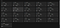The operating status of 26 IBM real Quantum computers. The quantum computers are accessible from the IBM Cloud (as of May 12, 2021).

This article discusses quantum bit (qubit), the basic computing unit of a Quantum computer.

# Classical and Quantum Computing

The approach in quantum computation is more likely to combine Classical and Quantum computers; thus, the term hybrid Classical-Quantum. It means part of the process is done in Classical computers, and another part of the process is done in Quantum computers.

A binary digit (bit)-based computer is a Classical computer, while a qubit-based computer is a Quantum Computer.

By having n-bits in the Classical computer, we choose one of the 2ⁿ values to do computation. E.g., the hex value 0x41 for 8 bits. However, by having n-qubits in the Quantum computer, we have all 2ⁿ values to do computation simultaneously.

# Quantum bit (Qubit)

We can build a physical qubit in many ways. For example, a qubit is constructed as an artificial atom in the IBM Quantum computer to promote quantumness.

Implementation is based on the superconducting qubit in the Josephson junction device, in which a current flows continuously without any voltage applied. It is achieved by having two superconducting materials separated by a thin insulating barrier operating at an extremely low temperature close to absolute zero, at 15 mK (milliKelvin).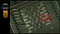A physical qubit realization in IBM Quantum chip, based on superconducting qubit (Zlatko Minev, 2020).

Other approaches exist, e.g., by creating qubits with trapped-ion, photonics, silicon-based, and others, including experiments at room temperature.

In this NISQ-era (Noisy Intermediate Scale Quantum), a qubit is not perfect (noisy). The quantum state can only be maintained (useful to do quantum computation) in the range of just tenths of microseconds before experiencing the decaying process called decoherence.

Take a look at the following illustration. The decoherence time (T1) of a 15-qubits IBM Quantum computer on the Cloud “ibmq_16_melbourne” is 54.27 microseconds. The quantum computer is online and operational when accessed on May 18, 2021.

`“The average T1 time of a qubit is the time it takes for a qubit to decay from the excited state to the ground state. It is important because it limits the duration of meaningful programs we can run on the quantum computer.,” stated in Qiskit Textbook (IBM, 2021b) within the section titled “Calibrating Qubits with Qiskit Pulse.”`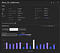The decoherence time (average T1 time) of a 15-qubits IBM Quantum computer on the Cloud “ibmq_16_melbourne”.

There are logical qubits and physical qubits. A logical qubit consists of many physical qubits. At present, a logical qubit needs to be built from hundreds to thousands of physical qubits, providing quantum error correction at the hardware level.

The qubit we refer to in this discussion (to do quantum computation) is the logical qubit.

# Bra-ket Notation

In Bra-ket notation, |Ψ> (Psi) represents a qubit state, which is the linear combination (superposition) of quantum states |0> and |1>.

• α, β are the coefficients of |0> and |1>
• α, β are the probability amplitudes, satisfying the equation |α|² + |β|² = 1
• |α|² and |β|² are the probability that the qubit state will collapse to 0 or 1 when measured, respectively
• α, β ∈ ℂ²

A quantum state Ψ in the form of <Ψ| is called a bra, while Ψ in the form of |Ψ> is called ket. Thus, the term Bra-ket notation. The following illustrates <Ψ| and |Ψ>, in which α and β are written in vector forms.

First of all, we do a qubit validity test by verifying that |α|²+|β|²=1.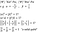Bra <Ψ| and ket |Ψ> notation, thus “Bra-ket” notation, 1 of 2.

Then, the ket |Ψ> and the bra <Ψ|.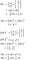Bra <Ψ| and ket |Ψ> notation, thus “Bra-ket” notation, 2 of 2.

A ket |Ψ> is a column vector, with values α and β . a bra <Ψ|is a row vector with values α*and β*. α* and β* are the complex conjugate of α and β. Complex conjugate means that we change the sign of the imaginary part of α and β from plus to minus, or vice versa. Then, we do a transpose operation following the complex conjugate operation — thus, completing the entire complex conjugate transpose operation.

As the sum of all probabilities is 1 (|α|² + |β|² = 1), the sum of all probabilities can also be expressed as α*α + β*β = 1.

By transforming a ket to a bra, we do complex conjugate of a column vector of a ket, then do matrix transpose — hence, performing a complex conjugate transpose. Vice versa, a bra to a ket transformation is done by doing complex conjugate of a row vector of a bra, then do matrix transpose.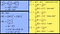Bra <Ψ| and ket |Ψ> notation, thus Bra-ket notation. Other possible quantum states are plus (|+>), minus (|->), i (|i>), and -i (|-i>).

The inner product of bra and ket is a measure of overlap between quantum states. An inner product of bra and ket, a row vector times the column vector, produces a single value, which is ∈ ℂ².

The inner product of the same quantum states is 1, while the inner product of the two orthogonal quantum states is 0.

The outer product of the ket and bra is a measure of projection. Typically, it is a projection to the z-axis (|0> and |1>, with probabilities defined by α² and β²). Projection to the z-axis is a projection to a computational basis (or a projection to standard basis).

An outer product of ket and bra, a column vector times the row vector, produces a matrix.

Projecting |Ψ> to |0> with probability |α|², as well as |Ψ> to |1> with probability |β|² are as follow:

Where α is the inner product of bra <0| and ket |Ψ>, while β is the inner product of bra <1| and ket |Ψ>.

In which, the projection matrices can be written as follows:

Let’s take a look at the following example in which the probability of measuring |0> is |α|² = 25%, and the probability of measuring |1> is |β|² = 75%. Thus, the probability amplitude α is 1/2, and the probability amplitude β is sqrt(3)/2. Since the imaginary part of both α and β is 0, for this case, α*=α and β*=β.

By measuring quantum state |0>, we have a 100% probability for getting 0 and a 0% probability for getting 1. Likewise, by measuring quantum state |1>, we have a 100% probability of getting 1 and 0% probability of 0.

When a qubit is in superposition, the probability of measuring 0 or 1 is equal at 50% and 50%, respectively.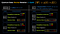When a qubit is in superposition, the probability of measuring 0 or 1 is equal at 50% and 50%, respectively.

# Bloch Sphere — Illustrating a 1-qubit state

A common approach to visually illustrate the state transition of a qubit is through a Bloch sphere. Mathematically, the state transition is performed by applying an operator to a qubit state.

A Bloch sphere is a mathematical construct of a 3 dimensions unit-ball in which a qubit with a polar coordinate |Ψ> = f(r, θ, ϕ) resides. Ψ is the function of r, theta (θ), and phi (ϕ). A unit ball has radius (r) equal to 1, so |Ψ> = f(r=1, θ, ϕ). θ is the angle (in radian) from the z-axis, while ϕ is the angle (in radian) from the x-axis. θ, ϕ ∈ ℜ.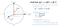The Atoms of Computation: The Bloch Sphere of a qubit (IBM, 2021b).

In general, we are projecting to the z-axis, which is a projection to |0> and |1>. However, we can have other projections such as to x-axis (a projection to |+> and |->), or y-axis (a projection to |i> and |-i>), and many more.

Translating the polar coordinate to cartesian, we have the following equations for x, y, and z. |Ψ> = f(r=1, θ, ϕ).

There are 6 commonly used quantum states |Ψ> for a given qubit state. |0> and |1>, |+> and |->, |i> and |-i>.

for z-axis, |0> and |1> :

for x-axis, |+> and |-> :

and for y-axis, |i> and |-i> :

The following animations illustrate various quantum state transitions of a qubit. Animations are generated using QuTiP libraries (P.D. Nation, J.R. Johansson, A.J.G. Pitchford, C. Granade, A.L. Grimsmo, N. Shammah, S. Ahmed, N. Lambert, B. Li, J. Lishman, and E. Giguère, 2021).

A quantum state transition from |0> to |1> :

• Initial state |Ψ> = |0> = f(r=1, θ=0, ϕ=0)
• Final state |Ψ> = |1> = f(r=1, θ=π, ϕ=0).

A quantum state transition from |1> to |0> :

• Initial state |Ψ> = |1> = f(r=1, θ=π, ϕ=0)
• Final state |Ψ> = |0> = f(r=1, θ=0, ϕ=0).

Quantum state transitions for Superposition, |0> to |+> and |1> to |-> :

|0> to |+>

• Initial state |Ψ> = |0> = f(r=1, θ=0, ϕ=0)
• Final state |Ψ> = |+> = f(r=1, θ=π/2, ϕ=0), a π/2 transition from θ=0

and |1> to |->

• Initial state |Ψ> = |1> = f(r=1, θ=π, ϕ=0)
• Final state |Ψ> = |-> = f(r=1, θ=3π/2, ϕ=0), a π/2 transition from θ=π/2.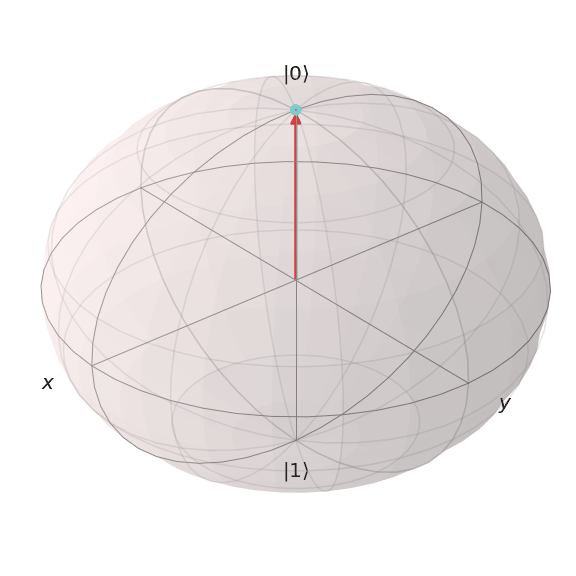Quantum state transitions for Superposition. |0> to |+> and |1> to |->.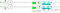An illustration of two independent qubits to illustrate Superposition: qubit 0 and qubit 1. The transition for qubit 0 is from state |0> to |+> (by applying H-gate), while qubit 1 from state |1> to |-> (also by applying H-gate). Illustrated by an online tool, Quirk (Strilanc, 2019).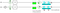An illustration of two independent qubits to illustrate Superposition: qubit 0 and qubit 1. The transition for qubit 0 is from state |0> to |+> (by applying H-gate), while qubit 1 from state |1> to |-> (also by applying H-gate). Note that, in order to bring qubit 0 to state |1>, an X-gate is applied to the |0> state). Illustrated by an online tool, Quirk (Strilanc, 2019).

Quantum state transitions from |+> to |-> and from |-> to |+> :

|+> to |->

• Initial state |Ψ> = |+> = f(r=1, θ=π/2, ϕ=0)
• Final state |Ψ> = |-> = f(r=1, θ=π/2, ϕ=π)

and |-> to |+>

• Initial state |Ψ> = |-> = f(r=1, θ=π/2, ϕ=π)
• Final state |Ψ> = |+> = f(r=1, θ=π/2, ϕ=0).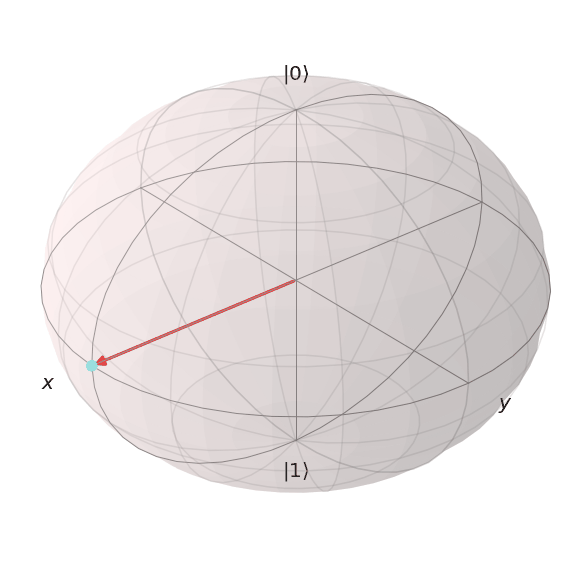Quantum state transitions from |+> to |-> and from |-> to |+>.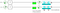An illustration of two independent qubits: qubit 0 and qubit 1. The transition for qubit 0 is from state |+> to |-> (by applying Z-gate, or Y-gate). Illustrated by an online tool, Quirk (Strilanc, 2019).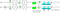An illustration of two independent qubits: qubit 0 and qubit 1. The transition for qubit 0 is from state |+> to |-> (by applying Z-gate, or Y-gate), while qubit 1 from state |-> to |+> (also by applying Z-gate, or Y-gate). Note that, in order to bring qubit 0 to state |+> and qubit 1 to |->, an H-gate is applied to both independently, by initially setting the state for qubit 0 = |0> and qubit 1 = |1>. Illustrated by an online tool, Quirk (Strilanc, 2019).

A quantum state transition from |i> to |-i> :

• Initial state = |Ψ> = |i> = f(r=1, θ=π/2, ϕ=π/2)
• Final state = |Ψ> = |-i> = f(r=1, θ=π/2 + π, ϕ=π/2).

A quantum state transition from |-i> to |i> :

• Initial state = |Ψ> = |-i> = f(r=1, θ=π/2 + π, ϕ=π/2)
• Final state = |Ψ> = |i> = f(r=1, θ=π/2, ϕ=π/2).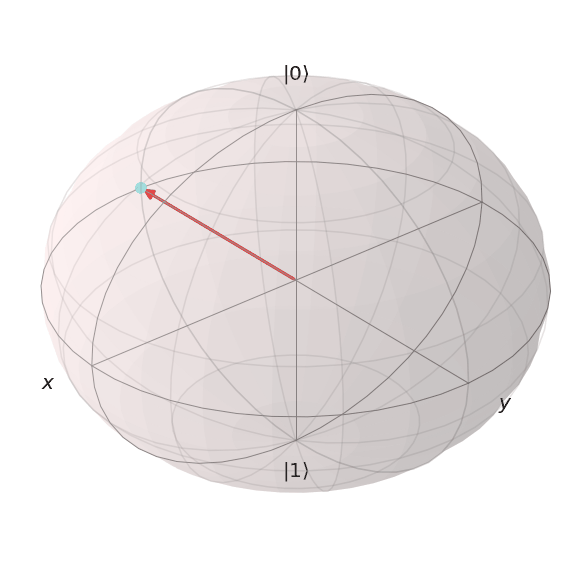Quantum state transitions from |-i> to |i>.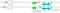An illustration of two independent qubits: qubit 0 and qubit 1. The transition for qubit 0 is from state |i> to -|i> (by applying Z-gate), while qubit 1 is from the state -|i> to |i> (also by applying Z-gate). Illustrated by an online tool, Quirk (Strilanc, 2019).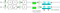An illustration of two independent qubits: qubit 0 and qubit 1. The transition for qubit 0 is from state |i> to -|i> (by applying Z-gate), while qubit 1 is from the state -|i> to |i> (also by applying Z-gate). Note that, in order to bring qubit 0 to state |+> and qubit 1 to |->, an H-gate followed by an S-gate (or S-gate inverse) are applied to both independently, by initially setting the states for both qubits = |0>. Illustrated by an online tool, Quirk (Strilanc, 2019).

# Measurement — with IBM Quantum

In a hybrid Classical-Quantum computing, a quantum program typically consists of the classical and the quantum part. Thus, the typical workflow will be as follows:

• A Classical program does necessary pre-processing, prepares a quantum circuit, then submits the quantum circuit as a “job” to a Quantum computer (or Quantum simulator) for execution using an API — Application Programming Interface (typically through a Cloud-based interface).
`Before execution, the submitted quantum circuit is translated to a series of hardware-specific supported basis gates for the specific Quantum computer. E.g., for the 27 qubits IBM Quantum computer called "ibmq_toronto" (IBM, 2021a), the supported basis gates are CX, ID, RZ, SX, and X. While for for the single qubit IBM Quantum computer called "ibmq_armonk", the supported basis gates are ID, RZ, SX, and X.`
• Quantum computer executes the translated Quantum circuit for some defined shots. E.g., 1024 shots (running the Quantum circuit 1024 times). Then return the measurement results to a Classical program.
• The Classical program reads the measurement results, then does necessary post-processing.

The following illustrates the measurement results of a qubit in superposition using a Quantum simulator. Measurement results show a 50% probability of the qubit collapse to 0 and a 50% probability for the qubit to collapse to 1. Thus, a perfect balance of 50%:50% to measure 0 and 1.

When we use a real Quantum computer to measure a qubit in superposition (e.g., with a 5-qubits Quantum Computer: “ibmq_santiago”), it shows that a perfect balance of 50%:50% measurement result is not there anymore (for the qubit to collapse to 0 and 1).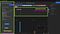A qubit in Superposition, executed in a real IBM Quantum computer, a 5-qubits “ibmq_santiago.”

There are slight variations in the measurement results. The impact of noisy qubit when running our Quantum circuit on today’s real Quantum computer. The measurement shows 569:455 frequency distribution (55.57%:44.43%) out of 1024 total shots for the probability to measure 0 and 1.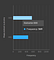Measurement frequency results for a qubit in Superposition, executed in a real IBM Quantum computer, a 5-qubits “ibmq_santiago.”

As additional information, mathematically, the state transition for qubits is achieved by applying an operator to qubit(s). Operators can be a NOT gate, a CNOT gate, an H-gate, or many others.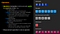Example of Operators. For manipulating quantum state transitions on qubits in a quantum circuit (IBM, 2021c).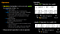Operators are implemented mathematically as matrices operations (IBM, 2021c).

# Moving Forward

IBM has an end-to-end Quantum technology accessible through the IBM Qiskit quantum framework (open source) since May 2016. IBM Quantum hardware is based on Superconducting technology and accessible from IBM Quantum Experience on the cloud or purchased separately as IBM Q System One. In addition, scalable “multiple quantum chips” targeted millions of qubits will be based on Condor quantum chip (1,121 qubits) targeted for release in 2023 (IBM Research, 2021).

In 2021, Qiskit also started to support IonQ hardware. In addition, there is also support for Qiskit Machine Learning for Artificial Intelligence, Qiskit Nature for Quantum Chemistry.

Qiskit Runtime has recently been introduced to provide a significant speedup by reducing non-necessary communications between the classical and the quantum computer in a hybrid classical-quantum environment, bringing the quantum circuits nearer to the Quantum computers.

Towards the end of 2021, upcoming articles in this Qubit Intuition series will cover topics such as Inner Product, Outer Product, & Tensor Product, Quantum Measurement, Unitary Matrices, Quantum Circuit & Reversible Transformation, as well as Quantum Algorithms.